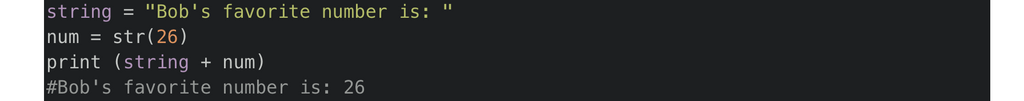# Python - Convert int to stringconvert int to string, python, python int to string, repr(), str()

One of the most common Python programming questions is, how do I convert strings to integers or integers to strings? Python int to string is a very common conversion. Check out our examples below to learn how to convert int to string using Python. If you need to convert bytes to string, have a look at our other Python post.

### Convert int to string using str()

The str() method is used for computing the “informal” string representation of an object. Simply put, str()’s objective is to be readable while the repr()'s goal is to be unambiguous. For example, if we suspect a float has a small rounding error, repr will show us while str may not.

```string = "Bob's favorite number is: "
num = str(26)
print (string + num)#Bob's favorite number is: 26```

### Convert int to string using repr()

The repr() method is used to compute the “official” string representation of an object. This representation has all information about the object, which is ideal when debugging etc. For printing the object use the str() method as mentioned above.

```num3 = repr(96)
print (string + num3)
#Bob's favorite number is: 96```

### Convert int to string using the backtick (“)

Backticks are a deprecated alias for `repr()`, the syntax was removed in Python 3.0. So don't try to use them unless you are on in Python 2.x. It is interesting to note that the backtick int to string cast seems to be faster than using `repr(num)` or `num.__repr__()` methods. The thought is that this is caused by the additional dictionary lookup that is required in the global namespace (for `repr`), or in the object's namespace (for `__repr__`).

```num2 = 21
print (string + `num2`)
#Bob's favorite number is: 21```

shop